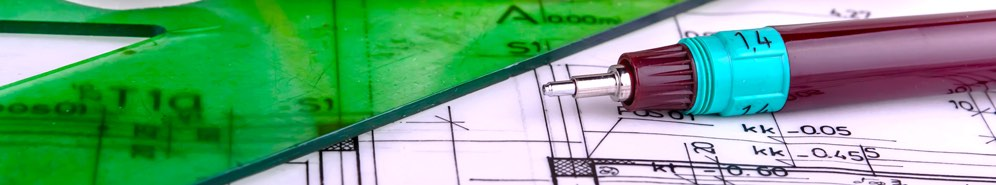Engineer Interview Questions in Boston, MA | Glassdoor# Engineer Interview Questions in Boston, MA

Engineers are responsible for designing and building products. In an interview context, expect to be quizzed on your technical skills, and also evaluated for your ability to work as a part of a team to get things built. The specific questions you are asked will depend on what type of engineering position you are looking for. Try a specific engineering discipline, such as software engineer, electrical engineer, or mechanical engineer.

## Top Interview Questions

Sort: RelevancePopular Date

### Software Engineer/Java Developer at BzzAgent was asked...

Dec 17, 2012
 Lots of questions about arrays, lists and hashmaps1 AnswerFocus more on how hashmap works and its applications

### Entry Level Software Engineer at General Dynamics Mission Systems was asked...

Mar 9, 2010
 What is an abstract class and why would you use it?2 AnswersIt's used to subclassing.In Java, an abstract class is a class which contains abstract methods, which are methods with declarations but no implementations. The methods are implemented by sub-classes of the abstract class. This gives more flexibility to those methods making it easier to tailor them to your use.

### Product Design Engineer at Apple was asked...

Mar 19, 2015
 If you have a cantilevered beam with a load at the unsupported end, what can you change about the beam to mitigate the effect of the load.2 AnswersReduce the length, increase the thickness, change the material to one with a stronger young's modulus (just have to be careful about the tradeoff between material density and stiffness).Taper it.

### Entry Level Software Engineer at General Dynamics Mission Systems was asked...

Jan 27, 2016
 Explain the development process of a previous project.1 AnswerI began with wireframing and worked forward through the mysql database, java backend, and the javascript and html front end. (I had a whiteboard to draw out my process)

### QA Automation Engineer at State Street was asked...

May 7, 2015
 what automation tool you have been using for your last project1 AnswerHP uft, LR, Selenium, badboy

### Java Software Engineer at Help Scout was asked...

May 24, 2016
 Write a method to determine if a string starts with an uppercase letter.1 Answerwouldn't it just be return string.charAt(0) == string.charAt(0).toLowerCase()

Jan 21, 2010

### Software Engineer at TripAdvisor was asked...

Apr 19, 2011
 Given four coins that could have any value (i.e. \$.17, \$.07, \$.05, \$.01), come up with an algorithm that figures out the fewest number of coins to get that value.6 AnswersBuild a tree where the root node value is the amount you are trying to solve. Off of that node make a new node for for the amount with the value of each coin subtracted off. Do this recursively until until all solutions are found then do a breadth first search until you get to a leaf.for each coin x=value of coin int q=x/25 int d=(x%25)/10 int n=((x%25)%10)/5 int p=((x%25)%10)%5 return (q+d+n+p)I think use dynamic programming to solve this problem is quite easy.Show More ResponsesIn Java: static int pickCoins(int valueIn, int[] coinsIn) { if (valueIn == 0) return 0; int num = 0; Set first = new HashSet(), second = new HashSet(), temp; first.add(valueIn); while (!first.isEmpty()) { num++; for (int i : first) { for (int j : coinsIn) { if (i-j == 0) return num; else if (i-j > 0) second.add(i-j); } // for-coinsIn } //for-first // swap first and second temp = second; first.clear(); second = first; first = temp; } //while return -1; } //pickCoins()public static int totalNum(int valueIn, int[] coinsIn){ Arrays.sort(coinsIn); int num = 0; for(int i = (coinsIn.length-1); i >= 0; i--){ num += valueIn/coinsIn[i]; valueIn = valueIn % coinsIn[i]; if (valueIn == 0) return num; } return -1; } From main: int[] coins = { 1, 7, 5, 11 }; int amount = 100;// \$1 tatalNum(amount, coins)Sorry. My description was a little ambiguous. I didn't mean using a quarter, dime, nickel, and penny. The coins themselves could have arbitrary values. For example, Coin A is worth 16 cents, Coin B is worth 9 cents, coin C is worth 4 cents, and coin D is worth 1 cent. Now using coins A, B, C, and D, find the fewest amount of coins to get to value X. Now the typical reaction is to subtract the largest coin from the total until the total is less then the value of that coin, repeat with the next smallest coin, and so on and count up the coins (like how the previous poster solved it). But that solution doesn't always work. Given the coin values described above, get the fewest coins for value X=18. This algorithm would result in 1 coin A and 2 coin Ds for a total of three coins but the correct answer is 2 coin Bs. The original solution with the tree and breadth first search is the way to get this answer.

Oct 17, 2011